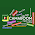# The horse, magnet and shoe puzzle answer.

The horse, magnet and shoe puzzle answer.

This puzzle is making waves on the internet. While some find it as a challenge to get the correct answer, those who are familiar with mathematics fines it easy.

Here's the puzzle in the screen shot below.

Solution:
Equation one.
3 horses = 30
Horse = 10.

Equation two
10 + 4 magnet = 18
4 magnet = 8.
Magnet = 2.

4 - pair of shoes = 2
- pair of shoes = -2
A pair of Shoes = 2.
If a pair of shoes= 2, implies a shoe = 1
Hence, Horse= 10,

Magnet = 2.

Shoe = 1.

Final equation.
I will substitute the values of horse, magnet and shoe in the  final equation and solve

A shoe + A horse × A magnet= ?
1 + 10 × 2= ?
1+ 20 = ?
21 = ?
1.1.